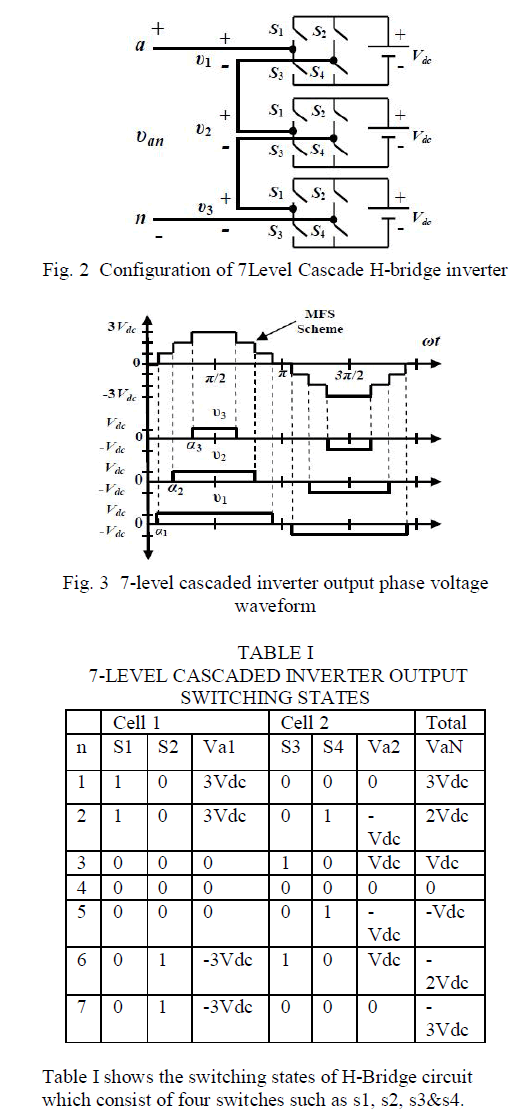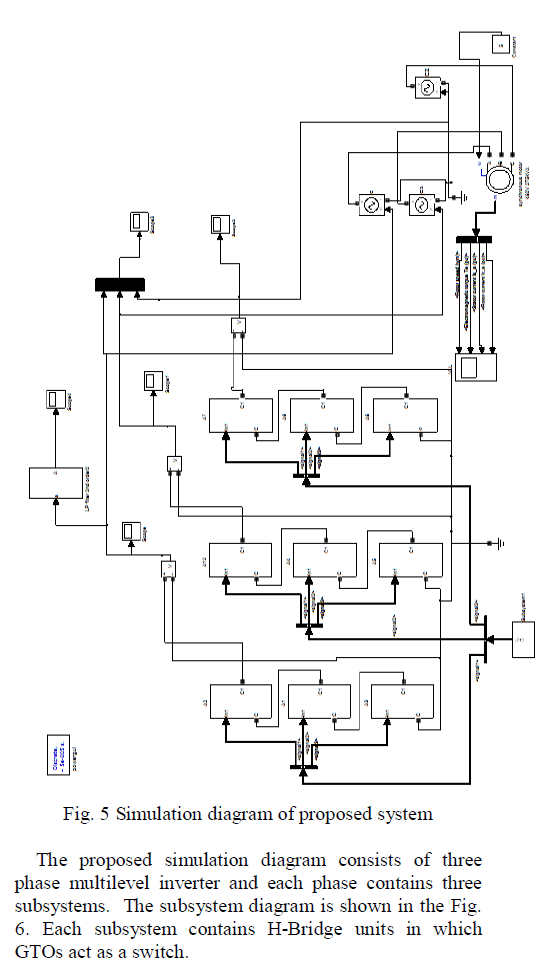ISSN ONLINE(2319-8753)PRINT(2347-6710)

All submissions of the EM system will be redirected to Online Manuscript Submission System. Authors are requested to submit articles directly to Online Manuscript Submission System of respective journal.

# Harmonic Minimization for Cascade Multilevel Inverter based on Genetic Algorithm

 Ranjhitha.G1, Padmanaban.K2 PG Scholar, Department of EEE, Gnanamani College of Engineering, Namakkal, India Assistant Professor, Department of EEE, Gnanamani College of Engineering, Namakkal, India Related article at Pubmed, Scholar Google

Visit for more related articles at International Journal of Innovative Research in Science, Engineering and Technology

## Abstract

Multilevel inverter have been attracting increasing interest recently due to the increased power rating, improving harmonic performance, and reduced electromagnetic inference (EMI) emission. In this project, a genetic algorithm (GA) optimization technique is applied to 7-level cascaded multilevel inverter which determines optimum switching angles to eliminate 5th and 7th order harmonics. Genetic Algorithm is developed as the preferred solution algorithm of specific harmonic elimination (PWMSHE) switching pattern. This project describes an efficient genetic algorithm that reduces significantly the computational burden resulting in fast convergence. The output voltage contains harmonics the different type of harmonics elimination methods are available. But all the method having some limitations. This project deals with how to reduce the particular harmonics in the output voltage of inverter. The concept of the harmonic stepped waveform technique for the multilevel inverter to be presented. By applying this concept, selected harmonic can be eliminated, and the output voltage THD can be improved. A procedure to achieve the appropriate switching angle is to be proposed. The proposed project is to be simulated by using MATLAB.

### Keywords

Multi level inverter, Selective harmonic elimination, Genetic algorithm, Total harmonic distortion

### INTRODUCTION

Nowadays high quality power is needed for medical, research and industrial applications to bring into being good quality results and for accurate evaluation. In this project, an attempt has been made to improve the quality of power. A seven level cascaded multi level inverter with identical dc supply is designed to reduce the harmonic components of the output voltage. Multilevel inverters continue to receive more and more attention because of their high voltage operation capability, low switching losses, high efficiency and low output of electromagnetic interference (EMI). The preferred output of a multilevel inverter is synthesized by several sources of dc voltages. With an increasing number of dc voltage sources, the inverter voltage waveform approaches a nearly sinusoidal waveform while using a low switching frequency scheme. This results in low switching losses, and because several dc sources are used to synthesize the total output voltage, each experiences a lower dv/dt compared to a single level inverter. Consequently, the multilevel inverter technology is a promising technology for high power electric devices such as utility applications. Selective Harmonic Elimination Pulse Width Modulation (SHE-PWM) has been intensively studied in order to achieve low THD. The common characteristic of the SHE-PWM method is that the waveform analysis is performed using Fourier theory.
The main objective of this project is to introduce a minimization technique assisted with Genetic Algorithm (GA) in order to reduce the computational burden associated with the solution of the nonlinear transcendental equations of the selective harmonic elimination method. An accurate solution is guaranteed even for a number of switching angles that is higher than other techniques would be able to calculate for a given computational effort.
The proposed system uses adaptive selective harmonic minimization method to minimize the harmonics in the case of cascade multilevel inverters. The cascaded multilevel inverter consists of a series of H-bridge inverter units. It consists of three H-bridges, each bridge module comprises of four Gate turn-offs Thyristor (GTO). Multilevel inverters offers several advantages such as, its capabilities to operate at high voltage, high efficiency, and low electromagnetic interference (EMI). Cascade Multilevel Inverter (CMLI) requires least number of components with compare to diode-clamped and flying capacitors type multilevel inverters and no specially designed transformer is needed as compared to multi pulse inverter. It has modular structure with simple switching strategy and occupies less space.
Selective Harmonic Elimination Pulse Width Modulation (SHE-PWM) has been intensively studied in order to achieve low THD. This method is to minimize the harmonics by determine the optimum switching angles for equal dc sources. Sets of non-linear transcendental equations are then derived using Fourier theory. The proposed approach is to choose the switching angles of both lower and higher order harmonics such as the 5th, 7th, 11th, and 13th are suppressed in the output voltage of the inverter.
A GA will be implemented to find the switching angles (offline) for a set of predetermined input voltages for a 7- level cascade inverter. We can avoid overhead and nosolution conditions by using the generalization shown in Fig. 2.ability of Genetic algorithm. Since GA runs fast, it is possible to quickly determine the switching angles to realize real-time control. The output of the multilevel inverter is fed to the second order low pass filter in order to reduce harmonic level and to get sinusoidal waveform.

### SEVEN LEVEL CASCADED H-BRIDGE INVERTER

The Cascade Multilevel Inverter (CMLI) is one of the most important topology in the family of multilevel and multi pulse inverters. It requires least number of components with compare to diode-clamped and flying capacitors type multilevel inverters and no specially designed transformer is needed as compared to multi pulse inverter. It has modular structure with simple switching strategy and occupies less space.
The cascaded H-bridges multilevel inverter is a relatively new inverter structure. It is proposed here to solve all the problems of the multilevel inverters as well as conventional multi pulse (PWM) inverters. This new multilevel inverter eliminates the excessively large number of
1) Bulky transformers required by conventional multi pulse inverters.
2) Clamping diodes required by multilevel diode clamped inverters.
3) Flying capacitors required by multilevel flying capacitor inverters.### MATHEMATICAL MODELS OF SWITCHING ANGLES AND SHE EQUATIONS FOR A CASCADE MULTILEVEL INVERTERdegree taking into consideration the quarter-wave symmetry of the output voltage waveform.
4. The most important item for the GA to evaluate the fitness of each chromosome is the cost function. The objective of this study is to minimize specified harmonics; therefore the cost function has to be related to these harmonics.
In this work the fifth and seventh harmonics at the output of a seven-level inverter are to be minimized. Then the cost function (f) can be selected as the sum of these two harmonics normalized to the fundamental,
f (Ө1, Ө 2, Ө 3) = 100(|V5| + |V7|)/V1
For each chromosome a multilevel output voltage waveform is created using the switching angles in the chromosome and the required harmonic magnitudes are calculated using FFT techniques.
The fitness value (FV) is calculated for each chromosome inserting. In this case,
FV (Ө1, Ө 2, Ө 3) = 100(|V5| + |V7|)/V1
The switching angle set producing the maximum FV is the best solution of the first iteration.
5. The GA is usually set to run for a certain number of iterations (100 in this case) to find an answer. After the first iteration, FVs are used to determine new offspring. These go through crossover and mutation operations and a new population is created which goes through the same cycle starting from FV evaluation. Sometimes, the GA can converge to a solution well before 100 iterations are completed. To save time, in this project, the iterations have been stopped when the absolute value of the cost function goes below 1, in which case the sum of the fifth and the seventh harmonics is negligible compared to the fundamental. Note that after these iterations, the GA finds one solution; therefore, it has to be run as many times as the number of solutions required to cover the whole modulation index range. The algorithm to find the optimum switching angles is described through the flow chart shown in Fig. 4.### CONCLUSIONS

The selective harmonic elimination method at fundamental frequency switching scheme has been implemented using the Genetic Algorithm that produces all possible solution sets when they exist. In comparison with other suggested methods, the proposed technique has many advantages such as: it can produce all possible solution sets for any numbers of multilevel inverter without much computational burden; speed of convergence is fast etc. The proposed technique was successfully implemented for computing the optimum switching angles for 7-level CMLI. A complete analysis for 7- level inverter has been presented and it is shown that a significant amount of THD reduction can be attained if all possible solution sets are computed.

### References

1. Adaptive Selective Harmonic Minimization Based on ANNs for Cascade Multilevel Inverters With Varying DC Sources, IEEE transactions on industrial electronics, vol. 60, no. 5, may2013, FaeteFilho, Member, IEEE, HelderZandonadi Maia, Tiago H. A. Mateus, BurakOzpineci, Senior Member, IEEE, Leon M.Tolbert, Senior Member, IEEE, and João O. P. Pinto, Member,IEEE

2. J. R.Wells, B.M. Nee, and P. L. Chapman, “Selective harmonic control: A general problem formulation and selected solutions,”IEEE Trans. Power Electron., vol. 20, no. 6, pp. 1337–1345, Nov.2005.

3. W. Lenwari, M. Sumner, and P. Zanchetta, “The use of genetic algorithms for the design of resonant compensators for active filters,” IEEE Trans.Ind. Electron., vol. 56, no. 8, pp. 2852–2861, Aug. 2009.

4. M. S. A. Dahidah and V. G. Agelidis, “Selective harmonic elimination PWM control for cascaded multilevel voltage source converters: A generalized formula,” IEEE Trans. Power Electron., vol. 23, no. 4, pp. 1620–1630, Jul. 2008.

5. Y. Liu, H. Hong, and A. Q. Huang, “Real-time calculation of switching angles minimizing THD for multilevel inverters with step modulation,”IEEE Trans. Ind. Electron., vol. 56, no. 2, pp.285–293, Feb. 2009.

6. Z. Du, L. M. Tolbert, and J. N. Chiasson, “Active harmonic elimination for multilevel converters,” IEEE Trans. Power Electron., vol. 21, no. 2,pp. 459–469, Mar. 2006.

7. H. Taghizadeh and M. T. Hagh, “Harmonic elimination of cascade multilevel inverters with nonequal DC sources using particle swarm optimization,”IEEE Trans. Ind. Electron., vol. 57,no. 11, pp. 3678–3684,Nov. 2010

8. Roger C. Dugan, Mark F. McGranaghan, H. Wayne Beaty :Electrical Power Systems quality. New York : McGraw Hill, c1996

9. Wilson E. Kazibwe and Mucoke H. Senduala : Electric Power Quality Control Techniques. New York: Van Nostrand Reinhold, c1993

10. IssaBatarseh : Power Electronic Circuits. New York : John Wiley, c2004

11. An application of PSO technique for harmonic elimination in a PWM inverter from World Wide Web

12. Z. Du, L. M. Tolbert, J. N. Chiasson, and H. Li, “Low switching frequency active harmonic elimination in multilevel converters with unequal DC voltages,” in Conf. Rec. IEEE IAS Annu.Meeting, Oct. 2005, vol. 1,pp. 92–98.